cmake is a code project build process management tool. I want to talk about its logo.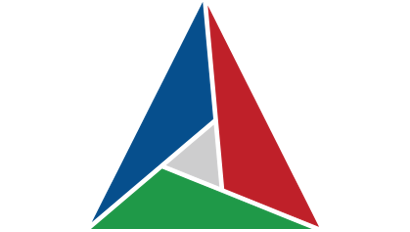This is their logo. If you can’t tell, the inner triangle is slightly off-center. This is what prompted me to see if I could recenter it.

To do so, I need to know how the original logo was made. By measuring it manually in Gimp, I noted the following things:

• The logo is not an equilateral triangle. However, it fits almost perfectely in a square, and is indeed isoceles
• The inner triangle is a fourth the size of the outer triangle
• Somehow, the inner triangle is rotated by 22.5° to the right, which is (90/4)°. This is likely to be a coincidence.

The fact that it isn’t an equilateral triangle puts the center of gravity of the inner triangle a bit to the left. If it were equilateral, it would be aligned and centered. So I decided to do just that.

This is the final result… or so I would like to say, but this was the final result when it was late at night and I wanted to get his post out as soon as possible.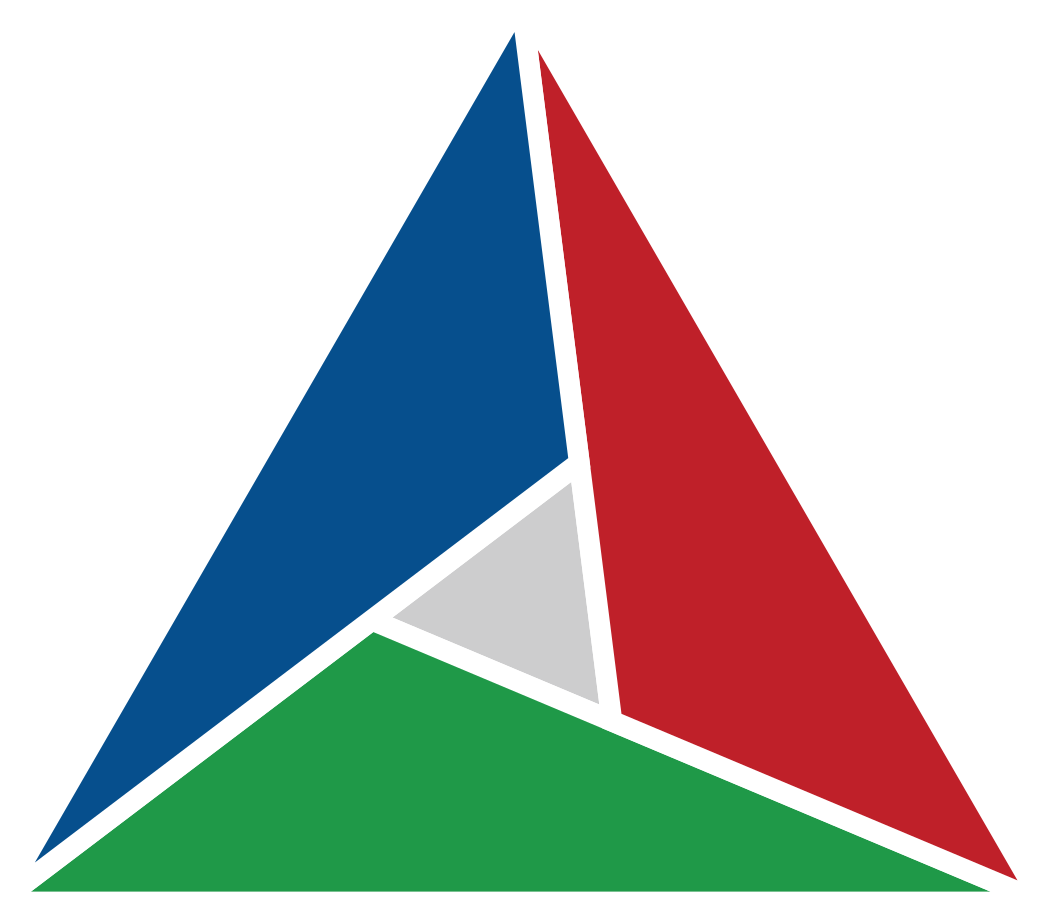This is what I ended up settling on.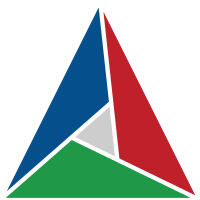Compared to the original, the gaps are a bit larger, but the inner triangle is indeed centered. The inner to outer ratio is still around a fourth give or take 10% (which is a lot). Also, the inner triangle is a bit smaller.

However, as it is not symmetrical, it doesn’t feel generic and keeps the character and feel it had originally. That’s something important to remember: “simple” perfection is boring.

I struggled a bit making it, so I’ll now detail how it was drawn. At first, I started making it equilateral, but the final step was to stretch it back to the original’s dimension.

## Solving the angle

First off, I decided to keep the inner to outer triangle ratio of 1 to 4. Since I couldn’t find a way to constrain the dimensions of the inner triangle based on the geometry like in CAD software, I had to calculate the positions of the points myself, or at least, the angles to use for the lines.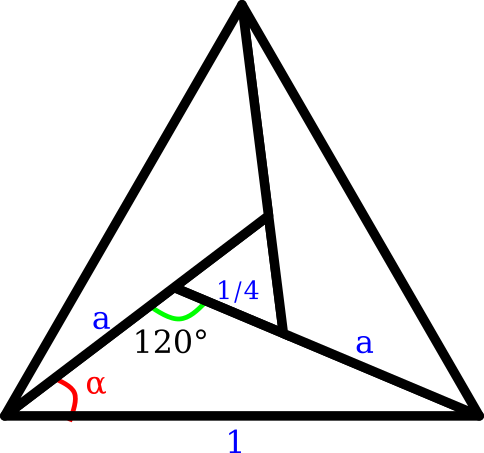In short, I had to solve this for a (the length of a connector from the outer to inner triangle) and α (the angle made by the left connector and the base):

We use the law of cosines also known as Al-kashi’s theorem:

$c^2 = a^2 + b^2 - 2ab\cos\gamma$

In our case, when applied to the green angle, gives us:

$1 = a^2 + \left( a + {1 \over 4} \right)^2 - 2 a \cos\left( { 2 \pi \over 3} \right)$

and with the red angle:

$\left( a + { 1 \over 4 } \right)^2 = a^2 + 1 - 2 a \cos \alpha$

Solving those two equations gives us $$a = ( \sqrt{21} - 1 ) / 8$$ and $$\alpha = \arccos \left( \left( a^2 + 1 - ( a + 1/4 )^2 \right) / 2a \right)$$.

## Generating a first svg

I then wrote a Perl script that would calculate those values and print out an initial svg to work with:

use v5.20;
use Math::Trig;

my $width = 1; my$height = sqrt(0.75);

my $aa = ( sqrt(21) - 1 ) / 8; my$alpha = acos( ( $aa**2 + 1 - (1/4 +$aa)**2 ) / ( 2*$aa ) ); # COLORS my$blue  = "#064f8d";
my $red = "#bf2029"; my$green = "#1f9948";
my $grey = "#cdcdce"; my %A = ( x =>$aa * cos($alpha), y =>$aa * sin($alpha), ); my %B = ( x => 1 -$aa * cos(pi/3 - $alpha), y =>$aa * sin(pi/3 - $alpha), ); my %C = ( x => ($aa + 0.25) * cos($alpha), y => ($aa + 0.25) * sin($alpha), ); say qq{<svg viewBox="0 0$width $height" xmlns="http://www.w3.org/2000/svg">}; say qq{ <polygon points="$A{x} $A{y},$B{x} $B{y},$C{x} $C{y}" fill="$grey"/>};
say qq{  <polygon points="0 0, $A{x}$A{y}, 1 0" fill="$green"/>}; say qq{ <polygon points="1 0,$B{x} $B{y}, 0.5$height" fill="$red"/>}; say qq{ <polygon points="0 0,$C{x} $C{y}, 0.5$height" fill="\$blue"/>};
say qq{</svg>};


The colors were taken from the original logo. I decided to create the shapes assuming a normal xy plane with x axis pointing right, and the y axis pointing up. This actually creates a vertically flipped image as the svg y axis points down.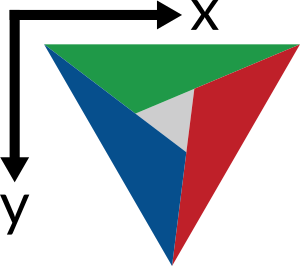In Inkscape, I then flip the image back upright.
As a side note, Inkscape really doesn’t like to zoom in when my image is a single pixel wide.

Although there is a version without gaps between the triangles, the one on the website has them.

I learned that you can create masks in Inkscape, which is using a grayscale image as a filter for another image. White means passthrough and black blocks display. Greys in between those two control how much is shown through the mask.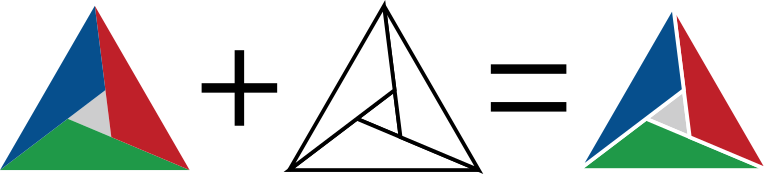Although that’s nice, it’s using a feature (mask) that might not supported by renderers as svg is actually really complex. So I want to “apply” the mask.

This can be done manually by using boolean operators. I’m too lazy to explain how they work. But remember that they only work on paths, so you should always convert your objects to paths before applying them.

## Exporting the svg

Inkscape has 3 ways to export svg: Inkscape svg, Plain svg, and Optimized svg.

Plain svg is a normal svg that usually contains some metadata and some inkscape extensions. An Inkscape svg also contains extra data that’s used solely by inkscape to keep track of the work. Optimized svg is a menu to produce an svg that is stripped of all the inkscape cruft.

If the difference between plain and inkscape svg is unclear, read more on the inkscape wiki.

Compare these two starts of files. The first one is inkscape svg, and the other is optimized svg.

<?xml version="1.0" encoding="UTF-8" standalone="no"?>
<svg
xmlns:dc="http://purl.org/dc/elements/1.1/"
xmlns:cc="http://creativecommons.org/ns#"
xmlns:rdf="http://www.w3.org/1999/02/22-rdf-syntax-ns#"
xmlns:svg="http://www.w3.org/2000/svg"
xmlns="http://www.w3.org/2000/svg"
xmlns:sodipodi="http://sodipodi.sourceforge.net/DTD/sodipodi-0.dtd"
xmlns:inkscape="http://www.inkscape.org/namespaces/inkscape"
viewBox="0 0 1 0.866025403784439"

<?xml version="1.0" encoding="UTF-8"?>
<svg version="1.1" viewBox="0 0 1 0.866025403784439" xmlns="http://www.w3.org/2000/svg">
<defs>
<polygon points=".35679 .27063 .58723 .17368 .55598 .42172" fill="#ffffff" stroke="#000000" stroke-width=".02"/>
<polygon points="0 0 .35679 .27063 1 0" fill="#ffffff" stroke="#000000" stroke-width=".02"/>
<polygon points="1 0 .58723 .17368 .5 .86603" fill="#ffffff" stroke="#000000" stroke-width=".02"/>
<polygon points="0 0 .55598 .42172 .5 .86603" fill="#ffffff" stroke="#000000" stroke-width=".02"/>
</defs>


## Final svg

After stretching it and exporting it optimized, this is the final svg source file:

<svg width="100" height="100" version="1.1" viewBox="0 0 100 100" xmlns="http://www.w3.org/2000/svg">
<path d="m51.719 5.8379l8.0078 73.4 37.898 18.412-45.906-91.813z" fill="#bf2029"/>
<path d="m54.754 53.475l-17.148 15.016 19.838 9.6387-2.6895-24.654z" fill="#cdcdce"/>
<path d="m35.852 70.027l-32.994 28.898h92.473l-59.479-28.898z" fill="#1f9948"/>
<path d="m49.328 3.7461l-45.922 91.842 51.062-44.723-5.1406-47.119z" fill="#064f8d"/>
</svg>


and here it is embedded in html: